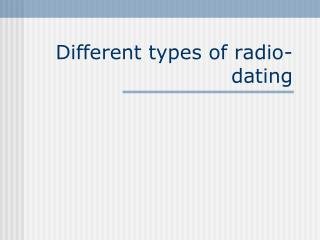DownloadDownload PresentationTélécharger la présentation- - - - - - - - - - - - - - - - - - - - - - - - - - - E N D - - - - - - - - - - - - - - - - - - - - - - - - - - -
##### Presentation Transcript

2. Radiodating • Radiodating always require you to determine the amount of radioactive isotopes present in the past and compare it to what is present today. • C-14 works because the amount of C-14 in living things is always the same. • The two types today will compare the amount of daughter isotope present to the amount of radioactive isotope to determine how old it is.

3. Potassium-40 dating • Rocks (never living) can also be dated if they have other certain isotopes. • K-40 decays into Ar-40. • When a rock is formed we can assume all gases would escape, so all argon in a rock should be the product of K-40 decay. • measure the K-40 and compare it to the Ar-40 and you can determine its age.

4. Uranium-238 dating • U-238 decays into Pb-206 which is extremely rare. • If you have a rock with U-238 and Pb-206 present, you can assume the Pb-206 came from the decay of U-238. • Scientists have come up with the 4.5 billion year age of the planet using these methods.

5. Math • The equation is difficult to use, so instead we will read it off a graph. • Here is equation mf/mi = 1/ 2hl • Percentage left is current mass/initial mass x100 % = mf/mi x 100 • Multiply the number of half lives by the value of one half life to get an age.

6. Problems • If you have 32% of a material left, how many half lives have passed? • 1.64 half lives • If you have 17% of Ra-223 left, how old is it? • 2.55 half lives x 11 days = • 28 days

7. Problems • If original sample had 78 g of Am-241, and you now have 53 g left; how old is the sample? • .56 half lives x 432 yrs • 240 years • A sample of Radon-222 is 9.4 days old. There are .27 g present, how much was originally present? • 1.5 g

8. Problems • If you have 15 g of Tc-96, and you assume you started with 88 g, how old is the object? • If you have an 8.0 day old sample of Radon-222 and there are 25 g present, how much was there to start?

9. Homework • If original sample had 28 g of Polonium-214, and you now have 13 g left; how old is the sample?